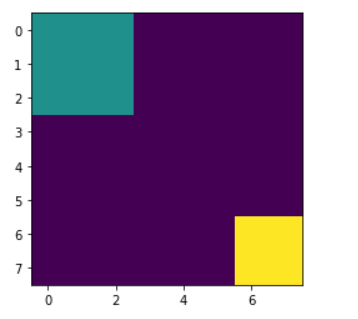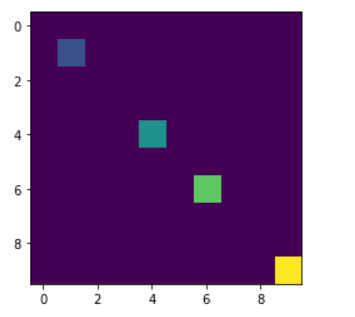# Mahotas – Weight of Labeled Region

In this article we will see how we can obtain weights of labeled region in mahotas. Labeled images are integer images where the values correspond to different regions. I.e., region 1 is all of the pixels which have value 1, region two is the pixels with value 2, and so on. By convention, region 0 is the background and often handled differently. We can create a labelled region with the help of `mahotas.label` method.

We can get the size of each region with the help of `mahotas.label_size` method, this size is measured simply as the number of pixels in each region. We can instead measure the total weight in each area.

In order to do this we will use `mahotas.label_size` method

Syntax : mahotas.labeled_sum(array, labeled_region)

Argument : It takes two numpy.ndarray object as argument i.e random array of region shape and labeled region

Return : It returns list of numpy.float64 values

Example 1:

 `# importing required libraries ` `import` `mahotas as mh ` `import` `numpy as np ` `from` `pylab ``import` `imshow, show ` ` `  `# creating region ` `# numpy.ndarray ` `regions ``=` `np.zeros((``10``, ``10``), ``bool``) ` ` `  `# setting 1 value to the region ` `regions[:``3``, :``3``] ``=` `1` `regions[``6``:, ``6``:] ``=` `1` ` `  `# getting labeled function ` `labeled, nr_objects ``=` `mh.label(regions) ` ` `  `# showing the image with interpolation = 'nearest' ` `imshow(labeled, interpolation ``=``'nearest'``) ` `show() ` ` `  `     `  `# random agaary of region shapes     ` `array ``=` `np.random.random_sample(regions.shape) ` ` `  `# getting sum i.e area ` `sums ``=` `mh.labeled_sum(array, labeled) ` ` `  ` `  `# printing the sums values ` `for` `i ``in` `range``(``len``(sums)): ` `    ``print``(``"Sum of region "` `+` `str``(i) ``+` `" : "` `+` `str``(sums[i])) `

Output :```Sum of region 0 : 38.81707025816505
Sum of region 1 : 5.627375253802732
Sum of region 2 : 8.224633573583985
```

Example 2:

 `# importing required libraries ` `import` `mahotas as mh ` `import` `numpy as np ` `from` `pylab ``import` `imshow, show ` ` `  `# creating region ` `# numpy.ndarray ` `regions ``=` `np.zeros((``10``, ``10``), ``bool``) ` ` `  `# setting 1 value in the region ` `regions[``1``, ``1``] ``=` `1` `regions[``6``, ``6``] ``=` `1` `regions[``4``, ``4``] ``=` `1` `regions[``9``, ``9``] ``=` `1` ` `  `# getting labeled function ` `labeled, nr_objects ``=` `mh.label(regions) ` ` `  `# showing the image with interpolation = 'nearest' ` `imshow(labeled, interpolation ``=``'nearest'``) ` `show() ` ` `  `     `  `# random agaary of region shapes     ` `array ``=` `np.random.random_sample(regions.shape) ` ` `  `# getting sum i.e area ` `sums ``=` `mh.labeled_sum(array, labeled) ` ` `  ` `  `# printing the sums values ` `for` `i ``in` `range``(``len``(sums)): ` `    ``print``(``"Sum of region "` `+` `str``(i) ``+` `" : "` `+` `str``(sums[i])) `

Output :

```Sum of region 0 : 47.05475409838963
Sum of region 1 : 0.07129110100184632
Sum of region 2 : 0.9333006775043519
Sum of region 3 : 0.8322767370999588
Sum of region 4 : 0.1700224211466459
```My Personal Notes arrow_drop_upCheck out this Author's contributed articles.

If you like GeeksforGeeks and would like to contribute, you can also write an article using contribute.geeksforgeeks.org or mail your article to contribute@geeksforgeeks.org. See your article appearing on the GeeksforGeeks main page and help other Geeks.

Please Improve this article if you find anything incorrect by clicking on the "Improve Article" button below.

Article Tags :

Be the First to upvote.

Please write to us at contribute@geeksforgeeks.org to report any issue with the above content.